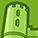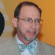cancel
Showing results for
Did you mean:New Contributor

## what is the mass

A wooden cube 20 cm in each direction is floating so that 46% of it is under water. How much mass does the block have?

Tags (1)Contributor III

## Re: what is the mass

Dear Audny,

That sounds a bit like a homework problem, so I will just outline the solution process and let you calculate the actual answer.

We know the density of water (1g/cm^3) and that buoyancy - flotation -  is the balance of an object's density with that of water.  SO, we first need to calculate the total volume of the block: 20cm x 20cm x 20cm.  If 46% of that volume is submerged, the mass submerged equals the mass of the same volume of water.  0.46 x 20cm x 20cm x 20cm will give you the grams of water displaced by the block, or the mass of the total block.

You could then use the original total volume and the calculated mass to calculate the density fo the block.

Best regards,

Steven